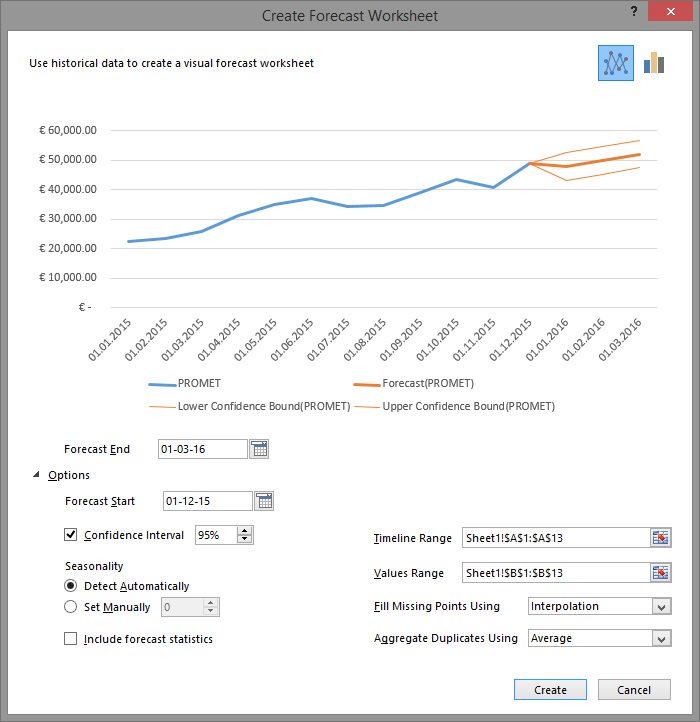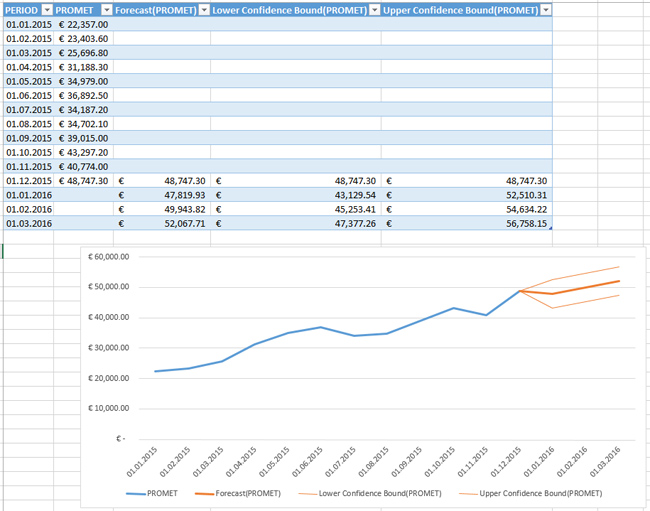# Forecast sheet

Forecast Sheet is an option used to calculate trends using specific forecasting functions. This is the Excel 2016 novelty. Based on the data provided for the appropriate time period, future values are forecasted and the lower and upper confidence interval is calculated. The calculated values are shown in the table along with a graph showing trends in data movement.

In order to use this option, we first need a data table. In this case, we will use one simple table with two columns. The first column contains dates for a period of one year. The second column shows the corresponding turnover values. First you need to select the data in the table, then go to the Data ribbon and run the Forecast Sheet option.On the screen, the Create Forecast Worksheet dialog box will appear, which at the very beginning will show the look of the future forecast chart. There are two icons in its upper right corner, by which we choose whether we want the chart to be displayed by lines (Line Chart) or columns (Column Chart). A line chart is probably more suitable for showing trends. In the lower left corner, there is the Options icon. Clicking on it opens the menu for detailed adjustment of forecasting options.The Forecast Start and Forecast End are used to enter the start date and end date of a forecast. Excel by automation calculates the end date, and for the start date it takes the first date from the data table. Values can be entered manually, and we can also choose them from a mini calendar that will open by clicking on the icon to the right of each of these fields.

The Confidence Interval option serves to choose whether we want to calculate the lower and upper confidence intervals. Also, we can state the percentage for this interval. The higher the percentage, the more secure are the data found in the initial table. This option is useful when we do not have complete data but we are looking at the sample. Then, we enter seasonality data (Seasonality). This is the number of intervals between two consecutive dates in the table. Basically, Excel offers a choice of Detect Automatically which means that it will evaluate seasonality itself, but we can also choose the Set Manually option and manually enter the number of intervals between two dates, which may in some cases increase the precision of the forecast. At the bottom of the window there is also the Include forecast statistics option. If it is selected, after calculating the prognosis, statistics will be shown showing how the results are displayed.

The Timeline Range and Values Range options are used to specify the columns in the table from which data needs to be retrieved. If in some cases it happens that we have incomplete data, it is useful to have set “option for filling the gaps” i.e. the Fill Missing points using. It helps us to determine whether we perform interpolation of data and which function we use for it (Agregate Duplicates using) or whether we want to replace the incomplete values with zeroes. By clicking on Create, based on the settings, we calculate the forecast and display the chart.